Anzeige

# Introductiontoflowchart 110630082600-phpapp01

19. Nov 2021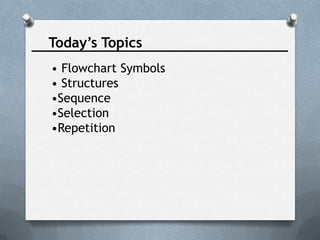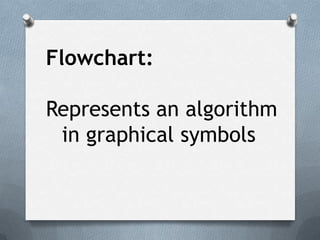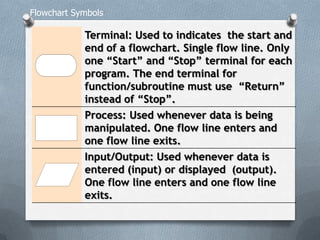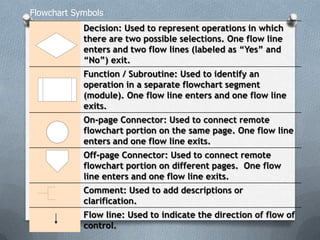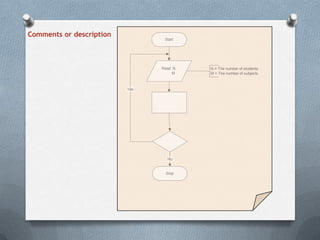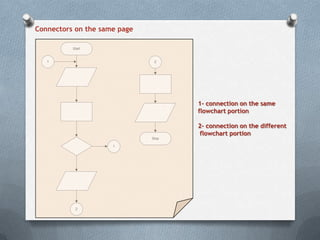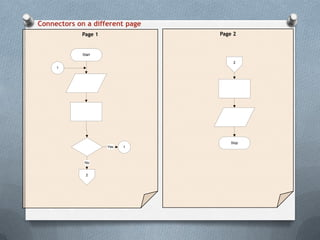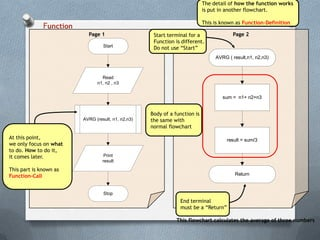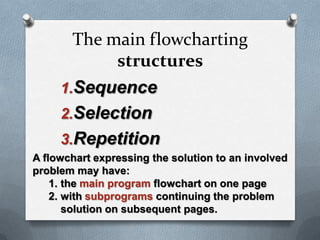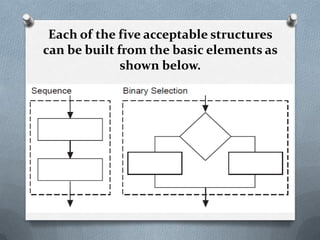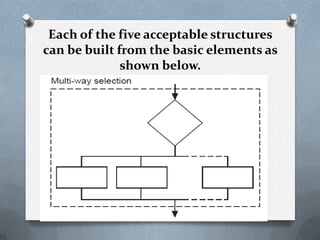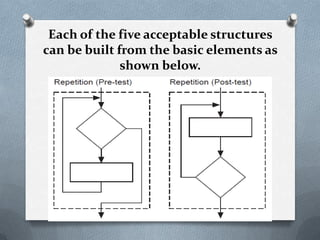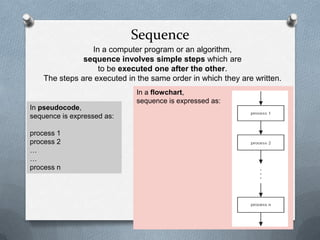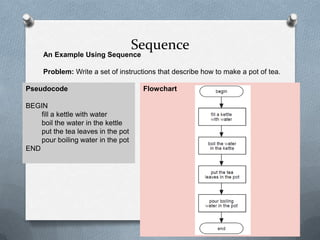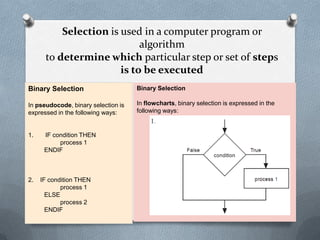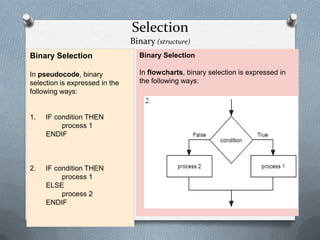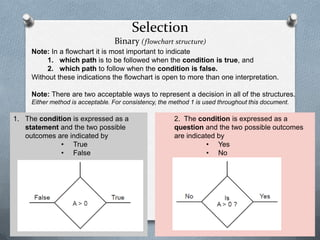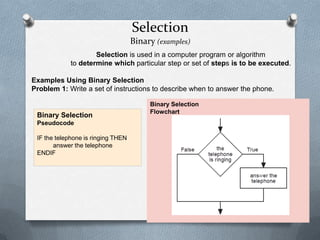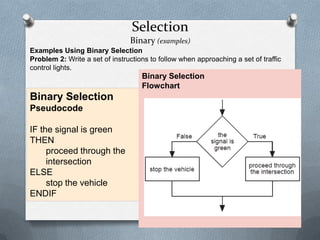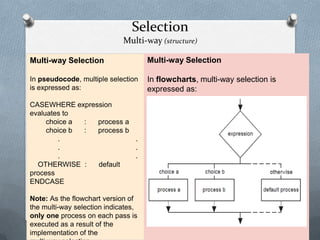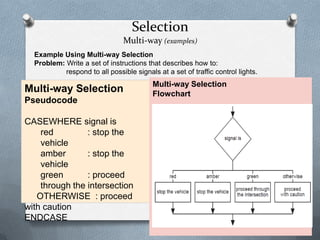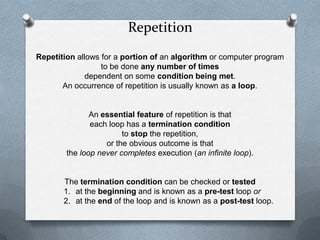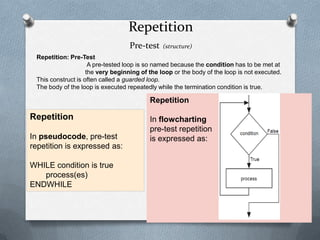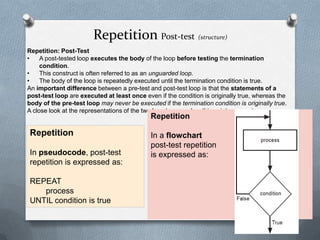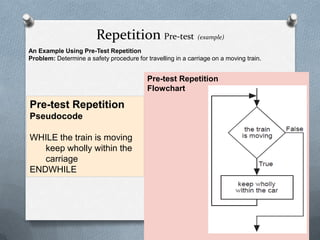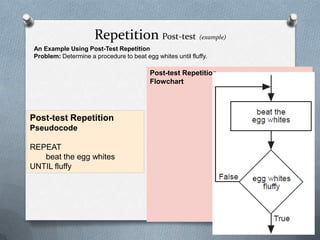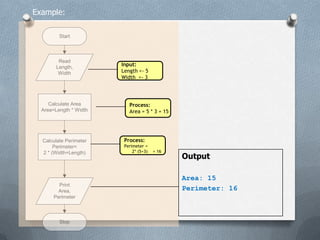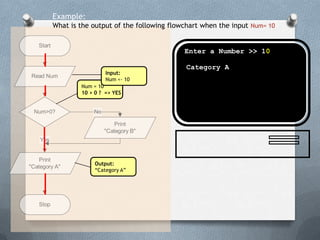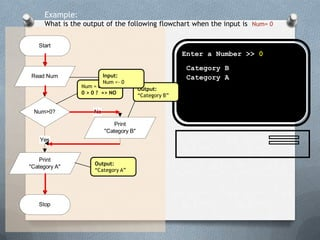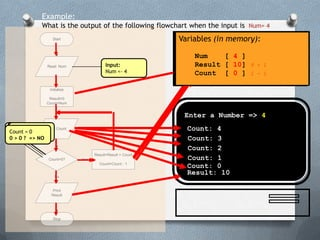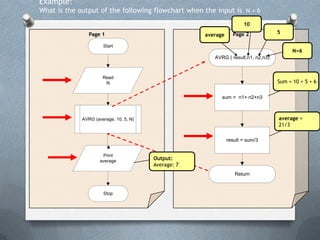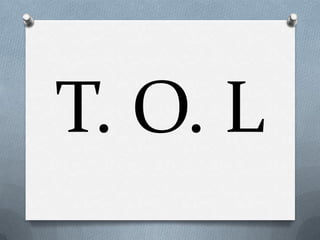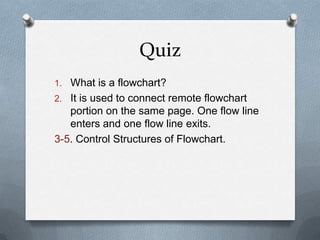1 von 34
Anzeige

### Introductiontoflowchart 110630082600-phpapp01

1. Introduction To Flowcharting
2. Today’s Topics • Flowchart Symbols • Structures •Sequence •Selection •Repetition
3. Flowchart: Represents an algorithm in graphical symbols
4. Terminal: Used to indicates the start and end of a flowchart. Single flow line. Only one “Start” and “Stop” terminal for each program. The end terminal for function/subroutine must use “Return” instead of “Stop”. Process: Used whenever data is being manipulated. One flow line enters and one flow line exits. Input/Output: Used whenever data is entered (input) or displayed (output). One flow line enters and one flow line exits. Flowchart Symbols
5. Decision: Used to represent operations in which there are two possible selections. One flow line enters and two flow lines (labeled as “Yes” and “No”) exit. Function / Subroutine: Used to identify an operation in a separate flowchart segment (module). One flow line enters and one flow line exits. On-page Connector: Used to connect remote flowchart portion on the same page. One flow line enters and one flow line exits. Off-page Connector: Used to connect remote flowchart portion on different pages. One flow line enters and one flow line exits. Comment: Used to add descriptions or clarification. Flow line: Used to indicate the direction of flow of control. Flowchart Symbols
6. Comments or description Start Read N, M No Yes Stop N = The number of students M = The number of subjects
7. Connectors on the same page Start 2 1 1 2 Stop 1- connection on the same flowchart portion 2- connection on the different flowchart portion
8. Connectors on a different page Page 1 Page 2 Start No 1 Yes 1 2 Stop 2
9. Function Page 1 AVRG (result, n1, n2,n3) Start Stop Read n1, n2 , n3 Print result Page 2 AVRG ( result,n1, n2,n3) Return sum = n1+ n2+n3 result = sum/3 End terminal must be a “Return” Start terminal for a Function is different. Do not use “Start” The detail of how the function works is put in another flowchart. This is known as Function-Definition At this point, we only focus on what to do. How to do it, it comes later. This part is known as Function-Call Body of a function is the same with normal flowchart This flowchart calculates the average of three numbers
10. The main flowcharting structures 1.Sequence 2.Selection 3.Repetition A flowchart expressing the solution to an involved problem may have: 1. the main program flowchart on one page 2. with subprograms continuing the problem solution on subsequent pages.
11. Each of the five acceptable structures can be built from the basic elements as shown below.
12. Each of the five acceptable structures can be built from the basic elements as shown below.
13. Each of the five acceptable structures can be built from the basic elements as shown below.
14. Sequence In a computer program or an algorithm, sequence involves simple steps which are to be executed one after the other. The steps are executed in the same order in which they are written. In pseudocode, sequence is expressed as: process 1 process 2 … … process n In a flowchart, sequence is expressed as:
15. Sequence An Example Using Sequence Problem: Write a set of instructions that describe how to make a pot of tea. Pseudocode BEGIN fill a kettle with water boil the water in the kettle put the tea leaves in the pot pour boiling water in the pot END Flowchart
16. Binary Selection In pseudocode, binary selection is expressed in the following ways: 1. IF condition THEN process 1 ENDIF 2. IF condition THEN process 1 ELSE process 2 ENDIF Binary Selection In flowcharts, binary selection is expressed in the following ways: Selection is used in a computer program or algorithm to determine which particular step or set of steps is to be executed
17. Selection Binary (structure) Binary Selection In pseudocode, binary selection is expressed in the following ways: 1. IF condition THEN process 1 ENDIF 2. IF condition THEN process 1 ELSE process 2 ENDIF Binary Selection In flowcharts, binary selection is expressed in the following ways:
18. Selection Binary (flowchart structure) Note: In a flowchart it is most important to indicate 1. which path is to be followed when the condition is true, and 2. which path to follow when the condition is false. Without these indications the flowchart is open to more than one interpretation. Note: There are two acceptable ways to represent a decision in all of the structures. Either method is acceptable. For consistency, the method 1 is used throughout this document. 1. The condition is expressed as a statement and the two possible outcomes are indicated by • True • False 2. The condition is expressed as a question and the two possible outcomes are indicated by • Yes • No
19. Selection Binary (examples) Selection is used in a computer program or algorithm to determine which particular step or set of steps is to be executed. Examples Using Binary Selection Problem 1: Write a set of instructions to describe when to answer the phone. Binary Selection Pseudocode IF the telephone is ringing THEN answer the telephone ENDIF Binary Selection Flowchart
20. Selection Binary (examples) Examples Using Binary Selection Problem 2: Write a set of instructions to follow when approaching a set of traffic control lights. Binary Selection Pseudocode IF the signal is green THEN proceed through the intersection ELSE stop the vehicle ENDIF Binary Selection Flowchart
21. Selection Multi-way (structure) Multi-way Selection In pseudocode, multiple selection is expressed as: CASEWHERE expression evaluates to choice a : process a choice b : process b . . . . . . OTHERWISE : default process ENDCASE Note: As the flowchart version of the multi-way selection indicates, only one process on each pass is executed as a result of the implementation of the Multi-way Selection In flowcharts, multi-way selection is expressed as:
22. Selection Multi-way (examples) Example Using Multi-way Selection Problem: Write a set of instructions that describes how to: respond to all possible signals at a set of traffic control lights. Multi-way Selection Pseudocode CASEWHERE signal is red : stop the vehicle amber : stop the vehicle green : proceed through the intersection OTHERWISE : proceed with caution ENDCASE Multi-way Selection Flowchart
23. Repetition Repetition allows for a portion of an algorithm or computer program to be done any number of times dependent on some condition being met. An occurrence of repetition is usually known as a loop. An essential feature of repetition is that each loop has a termination condition to stop the repetition, or the obvious outcome is that the loop never completes execution (an infinite loop). The termination condition can be checked or tested 1. at the beginning and is known as a pre-test loop or 2. at the end of the loop and is known as a post-test loop.
24. Repetition Pre-test (structure) Repetition: Pre-Test A pre-tested loop is so named because the condition has to be met at the very beginning of the loop or the body of the loop is not executed. This construct is often called a guarded loop. The body of the loop is executed repeatedly while the termination condition is true. Repetition In pseudocode, pre-test repetition is expressed as: WHILE condition is true process(es) ENDWHILE Repetition In flowcharting pre-test repetition is expressed as:
25. Repetition Post-test (structure) Repetition: Post-Test • A post-tested loop executes the body of the loop before testing the termination condition. • This construct is often referred to as an unguarded loop. • The body of the loop is repeatedly executed until the termination condition is true. An important difference between a pre-test and post-test loop is that the statements of a post-test loop are executed at least once even if the condition is originally true, whereas the body of the pre-test loop may never be executed if the termination condition is originally true. A close look at the representations of the two loop types makes this point apparent. Repetition In pseudocode, post-test repetition is expressed as: REPEAT process UNTIL condition is true Repetition In a flowchart post-test repetition is expressed as:
26. Repetition Pre-test (example) An Example Using Pre-Test Repetition Problem: Determine a safety procedure for travelling in a carriage on a moving train. Pre-test Repetition Pseudocode WHILE the train is moving keep wholly within the carriage ENDWHILE Pre-test Repetition Flowchart
27. Repetition Post-test (example) An Example Using Post-Test Repetition Problem: Determine a procedure to beat egg whites until fluffy. Post-test Repetition Pseudocode REPEAT beat the egg whites UNTIL fluffy Post-test Repetition Flowchart
28. Example: Start Stop Read Length, Width Print Area, Perimeter Calculate Area Area=Length * Width Calculate Perimeter Perimeter= 2 * (Width+Length) Input: Length <- 5 Width <- 3 Process: Area = 5 * 3 = 15 Process: Perimeter = 2* (5+3) = 16 Output Area: 15 Perimeter: 16
29. Start Stop Read Num Print "Category A" Yes Num>0? No Print "Category B" Example: What is the output of the following flowchart when the input Num= 10 Num = 10 10 > 0 ? => YES Input: Num <- 10 Enter a Number >> 10 Output: “Category A” Category A
30. Start Stop Read Num Print "Category A" Yes Num>0? No Print "Category B" Example: What is the output of the following flowchart when the input is Num= 0 Num = 0 0 > 0 ? => NO Output: “Category B” Input: Num <- 0 Enter a Number >> 0 Category B Output: “Category A” Category A
31. Start Stop Print Result Result=Result + Count Count=Count - 1 Initialize Result=0 Count=Num Count>0? Read Num No Print Count Yes Example: What is the output of the following flowchart when the input is Num= 4 Input: Num <- 4 Enter a Number => 4 Variables (in memory): Num [ ] Result [ ] Count [ ] Variables (in memory): Num [ 4 ] Result [ ] Count [ ] Count = 4 4 > 0 ? => YES Variables (in memory): Num [ 4 ] Result [ 0 ] Count [ 4 ] Count: 4 Variables (in memory): Num [ 4 ] Result [ 4 ] 0 + 4 Count [ 3 ] 4 - 1 Count: 3 Count = 3 3 > 0 ? => YES Variables (in memory): Num [ 4 ] Result [ 7 ] 4 + 3 Count [ 2 ] 3 - 1 Count: 2 Count = 2 2 > 0 ? => YES Variables (in memory): Num [ 4 ] Result [ 9 ] 7 + 2 Count [ 1 ] 2 - 1 Count: 1 Count = 1 1 > 0 ? => YES Variables (in memory): Num [ 4 ] Result [ 10] 9 + 1 Count [ 0 ] 1 - 1 Count: 0 Count = 0 0 > 0 ? => NO Result: 10
32. Page 1 AVRG (average, 10, 5, N) Start Stop Read N Print average Page 2 AVRG ( result,n1, n2,n3) Return sum = n1+ n2+n3 result = sum/3 Example: What is the output of the following flowchart when the input is N = 6 average 10 5 N=6 Sum = 10 + 5 + 6 average = 21/3 Output: Average: 7
33. T. O. L
34. Quiz 1. What is a flowchart? 2. It is used to connect remote flowchart portion on the same page. One flow line enters and one flow line exits. 3-5. Control Structures of Flowchart.
Anzeige